# 循环神经网络（Recurrent Neural Network）

## RNN

Recurrent neural networks 正是为了解决此类问题诞生的。RNN 网络连接中存在循环，并且能够存储信息。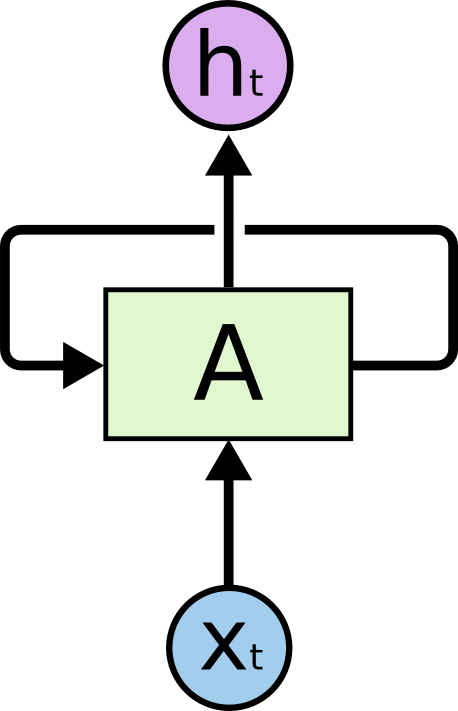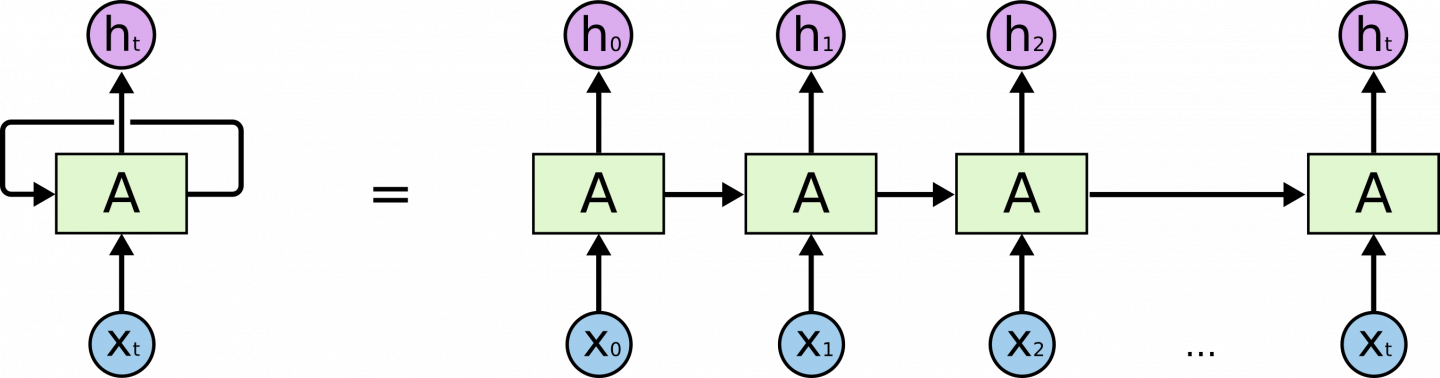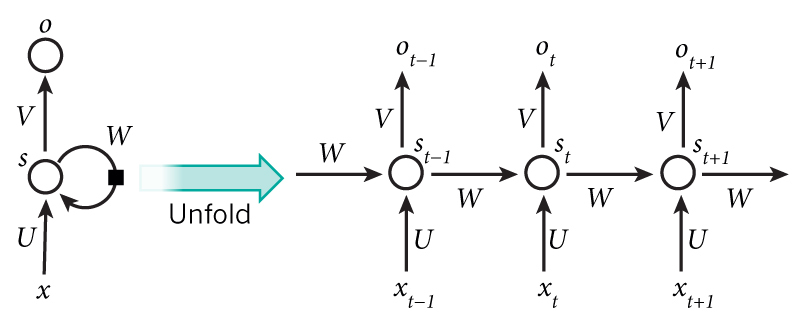• 1、xt：时间步 t 的输入向量，如 x1 可以是代表一句话（一个序列）第二个单词的 One-Hot 向量。
• 2、st：时间步 t 的输入向量 RNN 的隐藏状态（hidden state），即网络的记忆。st 计算依赖于上一个状态 st-1 和当前输入：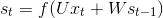。f 为非线性激活函数如 tanhReLU
• 3、ot（即上上图的 ht）：时间步 t 的输出，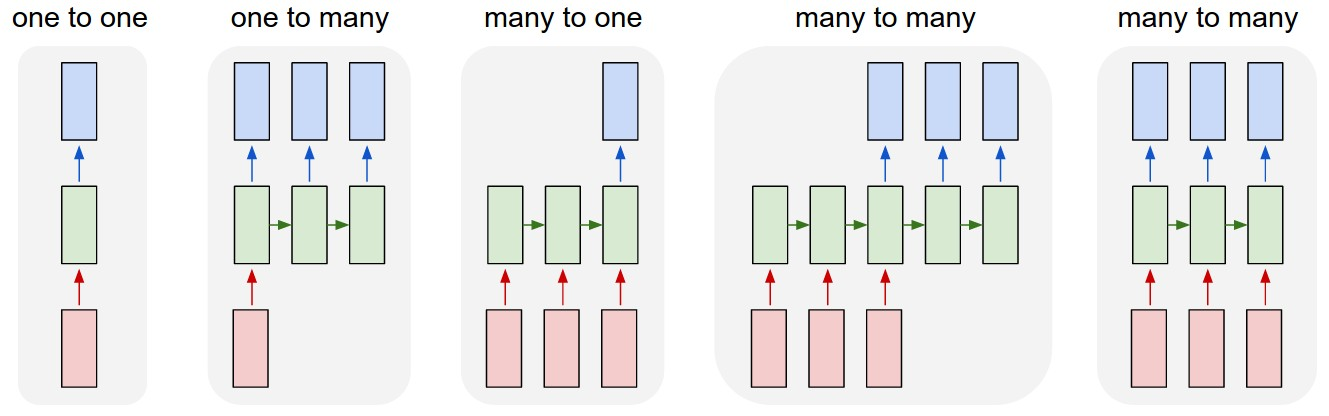• 1、是没有采用 RNN 的情形。如图像分类。
• 2、输入一个，输出一个序列（保留每个时间步的结果）。如输入一张图片，输出对这张图片的描述（Sequence）。
• 3、输入序列，只取最后一个时间步的结果。如输入一句话，来判断这句话的情感是消极的还是积极的，显然最后一个时间步结果最可靠，因为每个单词都进行了考虑。再比如，把一张图片看成序列，对图片分类，后面的例子就是用的这种。
• 4、输入序列，输出序列。如机器翻译，很好理解。
• 5、同步的（Synced ）序列输入和序列输出。如给视频的每一帧标上标签。

RNN 已经取得了很多成果：翻译，语音识别，语言建模，描述图片等，但普通的 RNN 很难训练，因为存在 梯度消失梯度爆炸 等问题。而 RNN 的变体，LSTM 正是为了解决此类问题诞生的。

## LSTM

LSTM，全称为长短期记忆网络(Long Short Term Memory Networks)，是一种特殊的RNN，能够学习到长期依赖关系。LSTM由Hochreiter & Schmidhuber (1997)提出，许多研究者进行了一系列的工作对其改进并使之发扬光大。LSTM在许多问题上效果非常好，现在被广泛使用。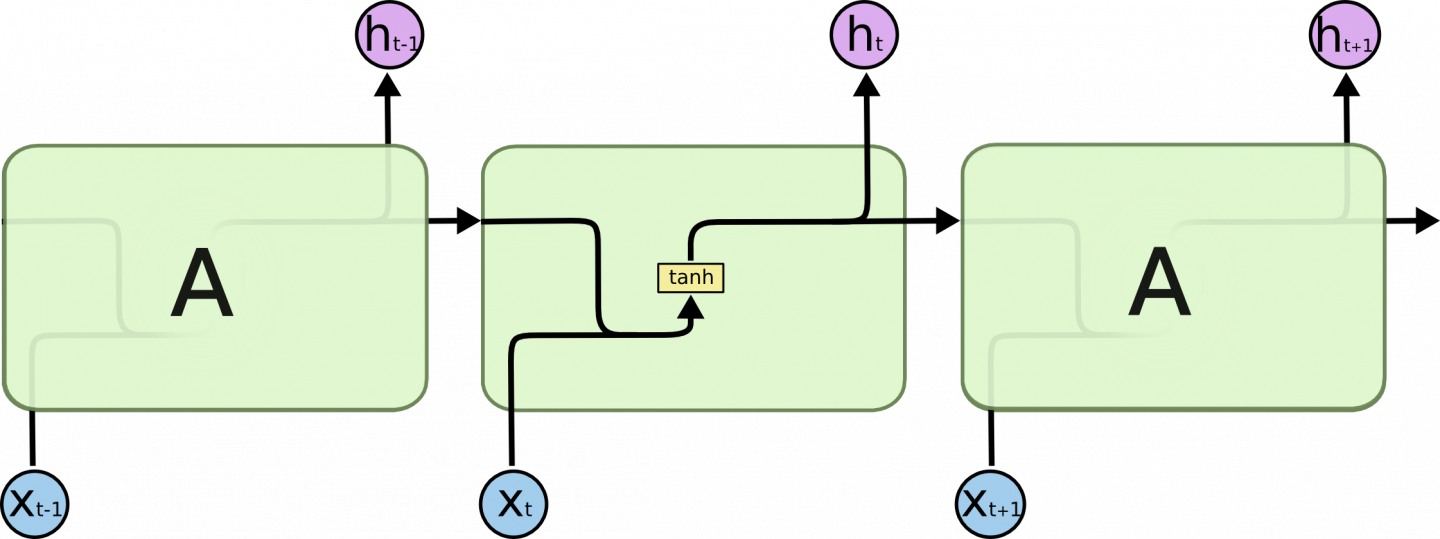LSTM 的整体结构（链式）与 RNN 一致，但 LSTM 内部有四层，并用一定的方式组织：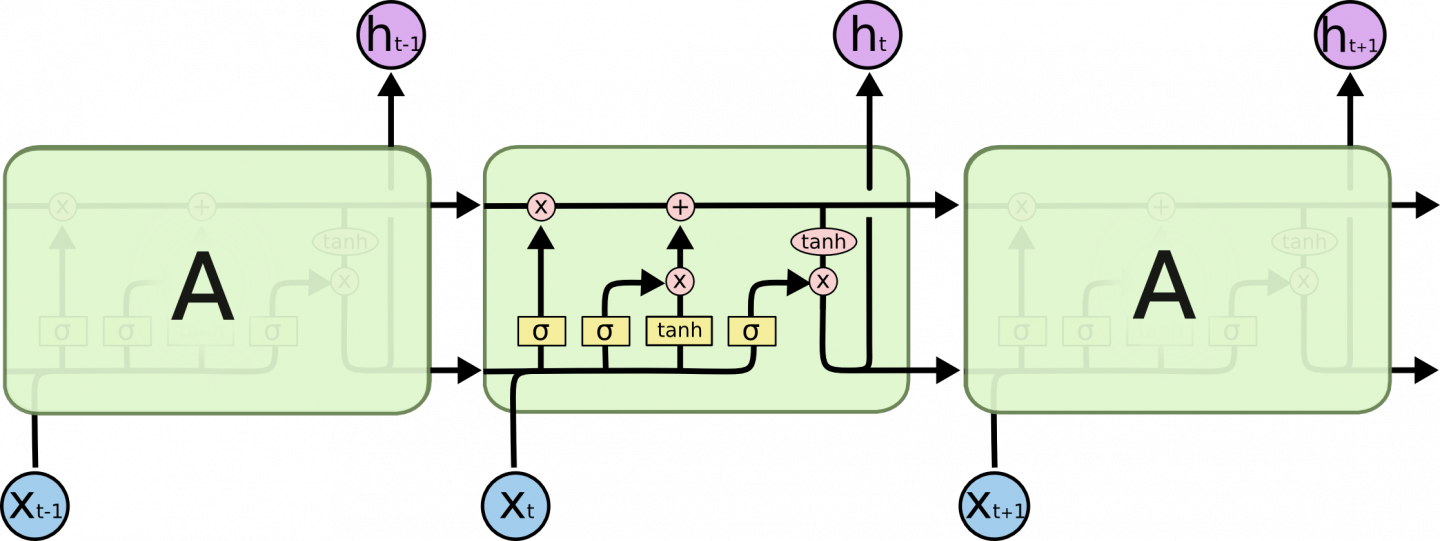LSTM 与 普通 RNN 的整体结构一样，都是输入 xt，输出 ot，传递给下一步 st。区别在于 st 的计算。普通 RNN st 的计算简单，而 LSTM 为了解决长期依赖关系，引入了三个“门”：Input, forget and output gates，因此计算也变得稍微复杂：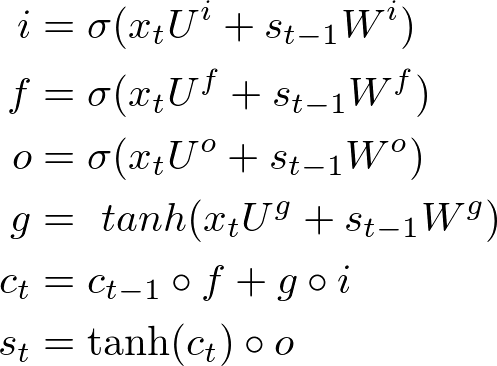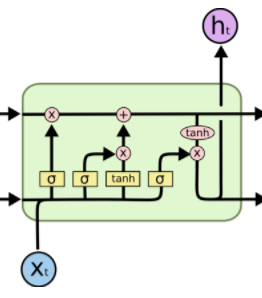## 用 LSTM 实现分类任务

RNN 解决了序列的依赖问题，如果我们把图片看成一个序列能对其完成分类吗？答案是肯定的。一张图片就是一个完整的序列，我们只取最后一个时间步的输出作为分类结果即可。

import tensorflow as tf
from tensorflow.contrib import rnn

# Import MNIST data
from tensorflow.examples.tutorials.mnist import input_data

mnist = input_data.read_data_sets("data/", one_hot=True)

lr = 0.001
training_iters = 100000
batch_size = 128
display_step = 10

n_input = 28
n_step = 28
n_hidden = 128
n_classes = 10

x = tf.placeholder(tf.float32, [None, n_step, n_input])
y = tf.placeholder(tf.float32, [None, n_classes])
w = tf.Variable(tf.random_normal([n_hidden, n_classes]))
b = tf.Variable(tf.random_normal([n_classes]))

def RNN(x):
# x (batch, n_step, n_input)
# x = tf.unstack(x, n_step, 1)
cell = rnn.BasicLSTMCell(n_hidden)

# static_rnn
# outputs: (n_step, batch, n_hidden)
# state: (batch, n_hidden), state is a tuple
# outputs, state = rnn.static_rnn(cell, x, dtype=tf.float32)
# -------------------------------------------------------
# outputs: (batch, n_step, n_hidden)
outputs, states = tf.nn.dynamic_rnn(cell, x, dtype=tf.float32)

return tf.matmul(tf.transpose(outputs, [1, 0, 2])[-1], w) + b

pred = RNN(x)

cost = tf.reduce_mean(
tf.nn.softmax_cross_entropy_with_logits(logits=pred, labels=y))

optimizer = tf.train.AdamOptimizer(lr).minimize(cost)
correct_pred = tf.equal(tf.argmax(pred, 1), tf.argmax(y, 1))
accuracy = tf.reduce_mean(tf.cast(correct_pred, tf.float32))

tf.summary.scalar('accuracy', accuracy)
tf.summary.scalar('loss', cost)
summaries = tf.summary.merge_all()

with tf.Session() as sess:
train_writer = tf.summary.FileWriter('logs/', sess.graph)
init = tf.global_variables_initializer()
sess.run(init)
step = 1
while batch_size * step < training_iters:
batch_x, batch_y = mnist.train.next_batch(batch_size)
batch_x = batch_x.reshape(batch_size, n_step, n_input)
sess.run(optimizer, feed_dict={x: batch_x, y: batch_y})
if step % display_step == 0:
acc, loss = sess.run(
[accuracy, cost], feed_dict={x: batch_x,
y: batch_y})
print("Iter " + str(step * batch_size) + ", Minibatch Loss= " + \
"{:.6f}".format(loss) + ", Training Accuracy= " + \
"{:.5f}".format(acc))
if step % 100 == 0:
s = sess.run(summaries, feed_dict={x: batch_x, y: batch_y})
train_writer.add_summary(s, global_step=step)

step += 1
print("Optimization Finished!")

test_len = 128
test_data = mnist.test.images[:test_len].reshape((-1, n_step, n_input))
test_label = mnist.test.labels[:test_len]
print("Testing Accuracy:", \
sess.run(accuracy, feed_dict={x: test_data, y: test_label}))

.............................................................
Iter 87040, Minibatch Loss= 0.107965, Training Accuracy= 0.96875
Iter 88320, Minibatch Loss= 0.169673, Training Accuracy= 0.96094
Iter 89600, Minibatch Loss= 0.115446, Training Accuracy= 0.96875
Iter 90880, Minibatch Loss= 0.106136, Training Accuracy= 0.96094
Iter 92160, Minibatch Loss= 0.199402, Training Accuracy= 0.96094
Iter 93440, Minibatch Loss= 0.061823, Training Accuracy= 0.98438
Iter 94720, Minibatch Loss= 0.132453, Training Accuracy= 0.95312
Iter 96000, Minibatch Loss= 0.044499, Training Accuracy= 1.00000
Iter 97280, Minibatch Loss= 0.161864, Training Accuracy= 0.94531
Iter 98560, Minibatch Loss= 0.114365, Training Accuracy= 0.95312
Iter 99840, Minibatch Loss= 0.127909, Training Accuracy= 0.96094
Optimization Finished!
Testing Accuracy: 0.992188

## 用 LSTM 实现回归任务

import tensorflow as tf
from tensorflow.contrib import rnn
import numpy as np
import matplotlib.pyplot as plt

BATCH_START = 0
TIME_STEPS = 20
BATCH_SIZE = 50
INPUT_SIZE = 1
OUTPUT_SIZE = 1
CELL_SIZE = 16
LR = 0.006

def get_batch():
global BATCH_START, TIME_STEPS
x = np.arange(BATCH_START, BATCH_START + BATCH_SIZE * TIME_STEPS).reshape(
(BATCH_SIZE, TIME_STEPS)) / (10 * np.pi)

sinx = np.sin(x)
cosx = np.cos(x)

BATCH_START += TIME_STEPS
return sinx[:, :, np.newaxis], cosx[:, :, np.newaxis], x

class RNN():
def __init__(self, x):
cell = rnn.BasicLSTMCell(CELL_SIZE)
self.cell_init_state = cell.zero_state(BATCH_SIZE, dtype=tf.float32)

outputs, self.final_state = tf.nn.dynamic_rnn(
cell, x, initial_state=self.cell_init_state, time_major=False)
outputs = tf.reshape(outputs, (-1, CELL_SIZE))
w = tf.Variable(tf.random_normal([CELL_SIZE, OUTPUT_SIZE]))
b = tf.Variable(tf.random_normal([OUTPUT_SIZE]))
self.pred = tf.matmul(outputs, w) + b

def ms_error(labels, logits):
return tf.square(tf.subtract(labels, logits))

X = tf.placeholder(tf.float32, [None, TIME_STEPS, INPUT_SIZE])
Y = tf.placeholder(tf.float32, [None, TIME_STEPS, OUTPUT_SIZE])
rnn = RNN(X)
pred = rnn.pred

cost = tf.reduce_mean(
tf.square(tf.subtract(tf.reshape(pred, [-1]), tf.reshape(Y, [-1]))))
optimizer = tf.train.AdamOptimizer(LR).minimize(cost)
correct_pred = tf.equal(tf.argmax(pred, 1), tf.argmax(Y, 1))
accuracy = tf.reduce_mean(tf.cast(correct_pred, tf.float32))

init = tf.global_variables_initializer()
sess = tf.Session()
sess.run(init)
plt.figure(figsize=(10, 5))
plt.ion()
plt.show()
for i in range(200):
sinx, cosx, x = get_batch()
if i == 0:
feed_dict = {
X: sinx,
Y: cosx,
}
else:
feed_dict = {
X: sinx,
Y: cosx,
rnn.cell_init_state: state,
}
_, state, outputs = sess.run(
[optimizer, rnn.final_state, pred], feed_dict=feed_dict)

plt.plot(x[0, :], cosx.flatten(), 'r', x[0, :],
outputs.flatten()[:TIME_STEPS], 'b--')
plt.ylim((-1.2, 1.2))
plt.xlim(x[0, 0] - 10, x[0, -1])
plt.draw()
plt.pause(0.1)

if i % 20 == 0:
print('cost: ', sess.run(cost, feed_dict=feed_dict))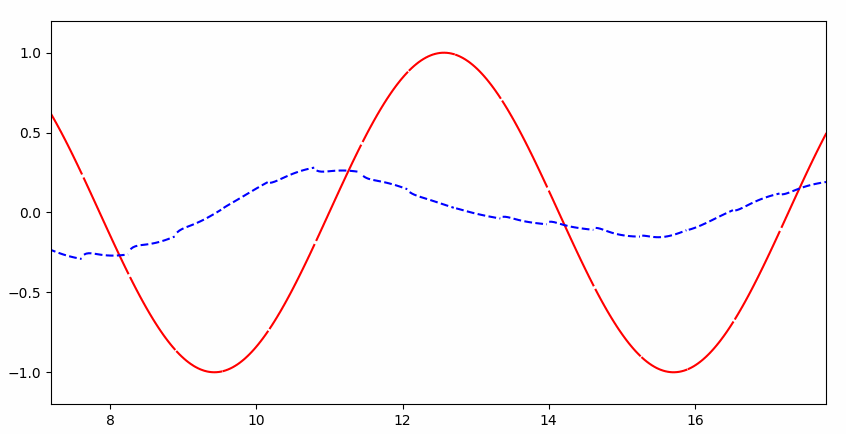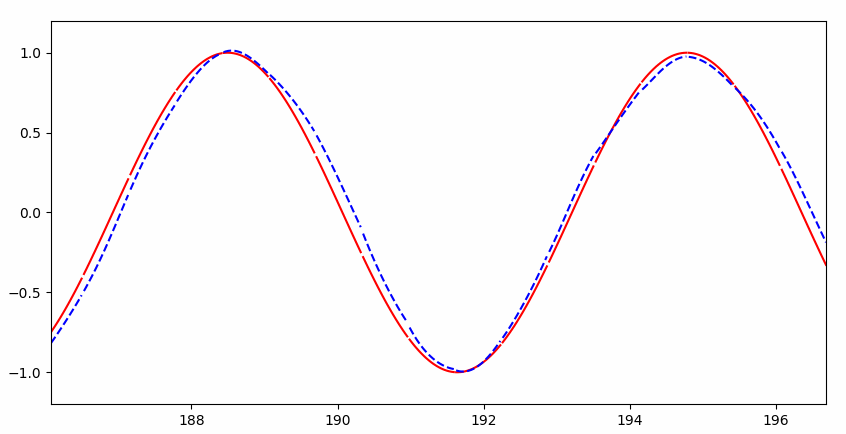## RNN 与普通神经网络的直观区别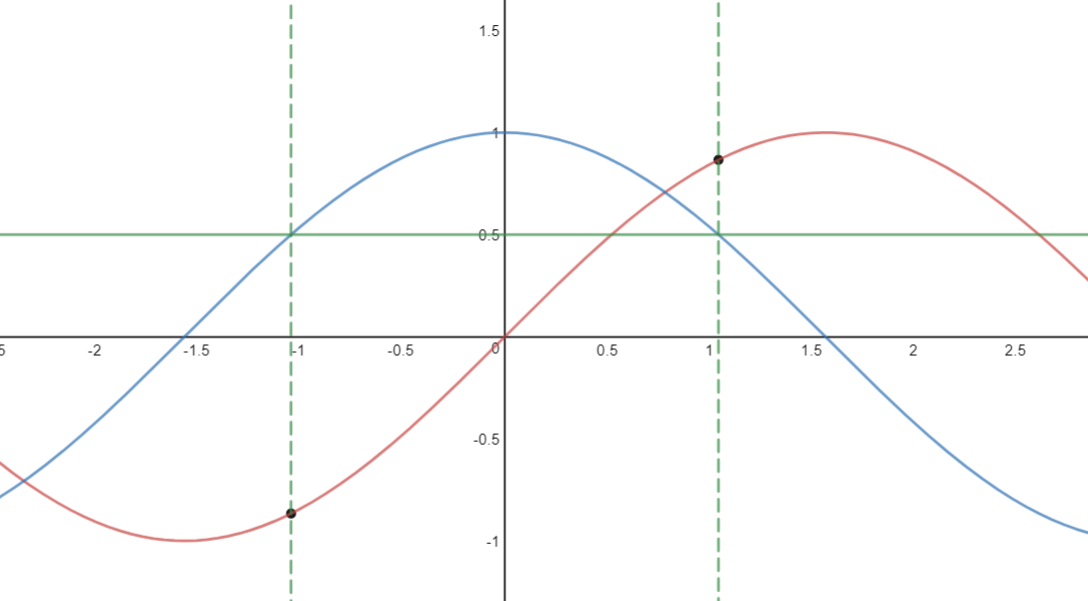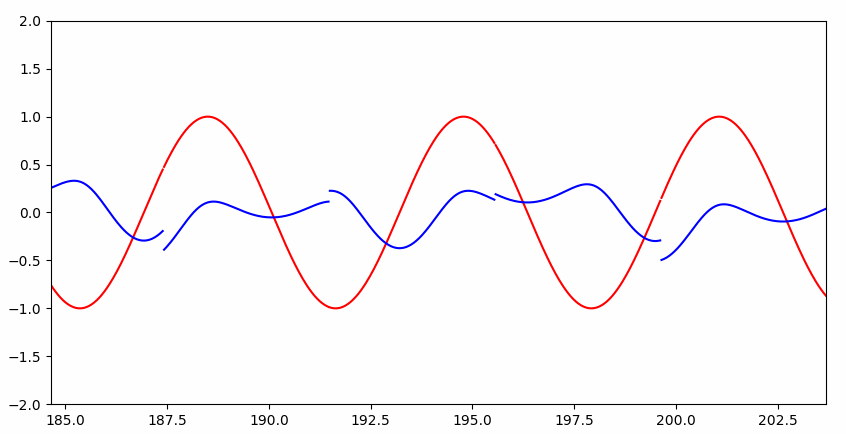## 参考

Understanding LSTM Networks
The Unreasonable Effectiveness of Recurrent Neural Networks